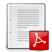# An Abstract Approach to Stratification in Linear LogicPhoto en By-Nc par whatsthatpicture

avec Damiano Mazza et Lorenzo Tortora de Falco

, par

We study the notion of stratification, as used in subsystems of linear logic with low complexity bounds on the cut-elimination procedure (the so-called light logics), from an abstract point of view, introducing a logical system in which stratification is handled by a separate modality. This modality, which is a generalization of the paragraph modality of Girard’s light linear logic, arises from a general categorical construction applicable to all models of linear logic. We thus learn that stratification may be formulated independently of exponential modalities ; when it is forced to be connected to exponential modalities, it yields interesting complexity properties. In particular, from our analysis stem three alternative reformulations of Baillot and Mazza’s linear logic by levels : one geometric, one interactive, and one semantic.

Version finale "preprint" d’un papier à paraître cette année.LLlevSem.pdf
An Abstract Approach to Stratification in Linear Logic (version preprint)

http://arxiv.org/abs/1206.6504